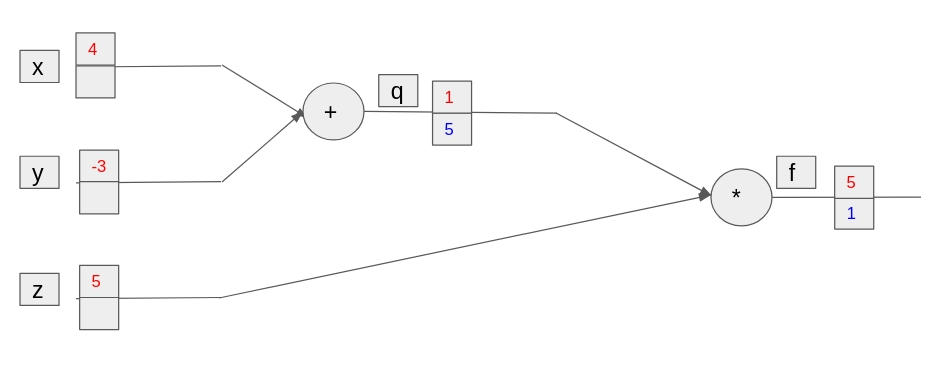# Backpropagation by handGiven the computational graph above, we want to calculate the derivatives for the leaf nodes (x, y and z). To get you started we already calculated the results of the forward pass (in red) in addition to calculating the derivatives of f and q.

The rules for derivative computations have been given in the table below:

Interaction Overall Change
Addition $$(f+g)' = f' + g'$$
Multiplication $$(f \cdot g)' = f \cdot dg + g \cdot df$$
Powers $$(x^n)' = \frac{d}{dx}x^n = nx^{n-1}$$
Inverse $$(\frac{1}{x})' = - \frac{1}{x^2}$$
Division $$(\frac{f}{g})' = (df \cdot \frac{1}{g}) + (\frac{-1}{g^2}dg \cdot f)$$